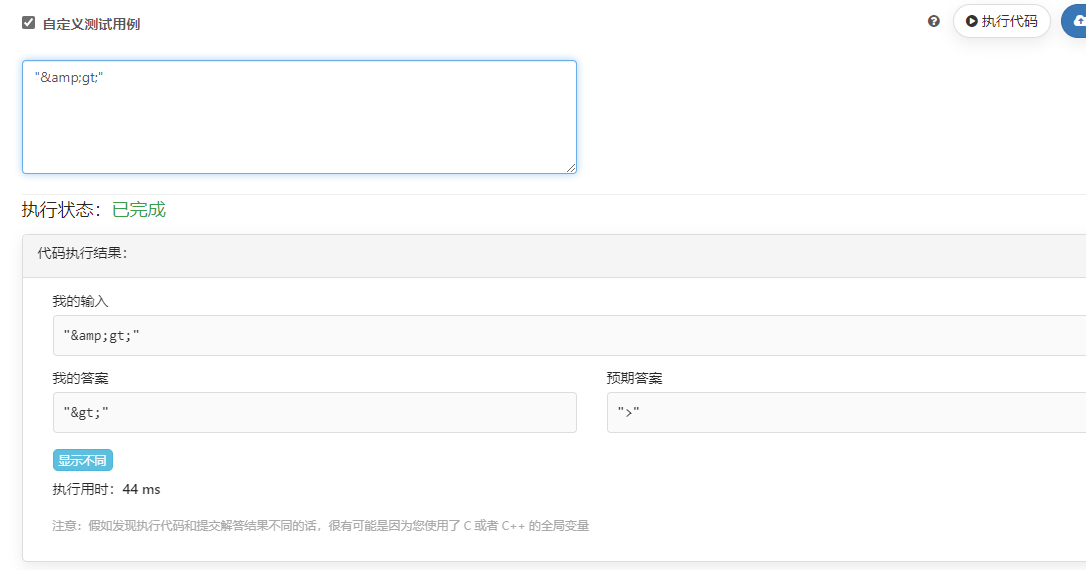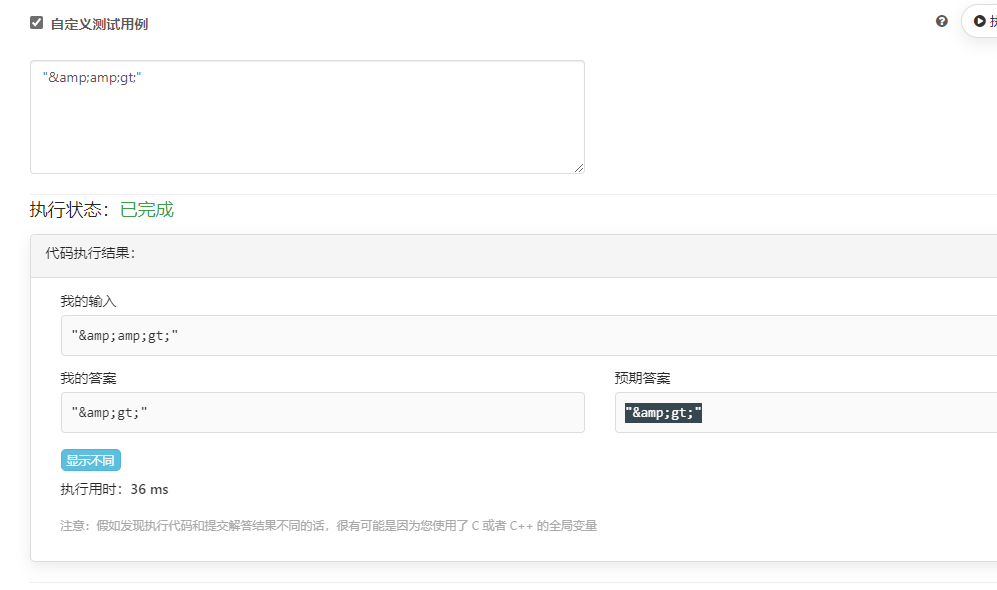🏆 第 184 场力扣周赛

python 题解 https://leetcode-cn.com/circle/article/oCBsqp/

## 5380.数组中的字符串匹配

class Solution:
def stringMatching(self, words: List[str]) -> List[str]:
ans = []
for i in range(len(words)):
for j in range(len(words)):
if i == j: continue
if words[i] in words[j]:
ans.append(words[i])
break
return ans


## 5381.查询带键的排列

class Solution:
def processQueries(self, queries: List[int], m: int) -> List[int]:
m = min(m, max(queries))
p = [i+1 for i in range(m)]
ans = []
for x in queries:
i = p.index(x)
ans.append(i)
x = p.pop(i)
p.insert(0, x)
return ans


## 5382.HTML 实体解析器

class Solution:
def entityParser(self, text: str) -> str:
text = text.replace('&quot;', '"')
text = text.replace('&apos;', '\'')
text = text.replace('&amp;', '&')
text = text.replace('&gt;', '>')
text = text.replace('&lt;', '<')
text = text.replace('&frasl;', '/')
return text


class Solution:
def entityParser(self, text: str) -> str:
text = text.replace('&quot;', '"')
text = text.replace('&apos;', '\'')
text = text.replace('&amp;', '&')
text = text.replace('&gt;', '>')
text = text.replace('&lt;', '<')
text = text.replace('&frasl;', '/')
return text## 5383.给 N x 3 网格图涂色的方案数

A 开头有：
ABA ABC ACA ACB
B 开头有：
BAB BAC BCA BCB
C 开头有：
CAB CAC CBA CBC

ABA 模式有 6 种：ABA ACA BAB BCB CAC CBC
ABC 模式有 6 种：ABC ACB BAC BCA CAB CBA

第一层   ABA   ABA   ABA   ABA   ABA
|||   |||   |||   |||   |||



第一层   ABC   ABC   ABC   ABC
|||   |||   |||   |||



MOD = 1000000007
aba2 = (aba * 3 + abc * 2) % MOD
abc2 = (aba * 2 + abc * 2) % MOD


aba 和 abc 初始都为 6

class Solution:
def numOfWays(self, n: int) -> int:
aba = 6
abc = 6
MOD = 1000000007
for _ in range(n-1):
aba2 = (aba * 3 + abc * 2) % MOD
abc2 = (aba * 2 + abc * 2) % MOD
aba, abc = aba2, abc2
return (aba + abc) % MOD

25